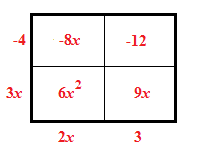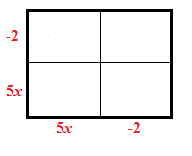### Home > AC > Chapter 8 > Lesson 8.2.1 > Problem8-50

8-50.

Use a generic rectangle to multiply each expression below.

1. $\left(3x-4\right)\left(2x+3\right)$

Use a generic rectangle to multiply.Multiply the dimensions of each smaller section to find the area of each tile piece.

Add together the tile pieces and add like terms to simplify the expression.
$6x^{^2}+9x-8x-12$
$6x^{^2}+x-12$

1. $\left(5x-2\right)^2$

Use a generic rectangle to simplify.